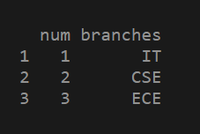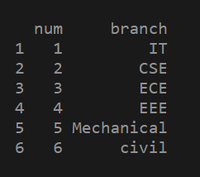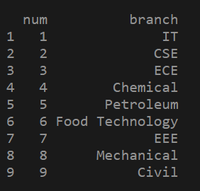Skip to content
Related Articles
Insert multiple rows in R DataFrame
• Last Updated : 01 Apr, 2021

In this article, we are going to see how to insert multiple rows in the dataframe in R Programming Language. First, let’s create a DataFrame

To create a data frame we need to use vectors. We need to create vectors with some values and pass the vectors into data.frame() function as parameter. Thus, a data frame is created. Let us see an example that demonstrates the above statement.

Example 1:

## R

 `# here num and branches are the vectors, ans those``# are passed into data.frame() as parameters .``dataframe = ``data.frame``(num=``c``(1:3),branches=``c``(``"IT"``,``"CSE"``,``"ECE"``))`` ` `# In this example we created vectors inside the``# data frame() function and assigned values to vectors. ``print``(dataframe)`

Output:In the above example, we have created a data frame with vectors directly as parameters. We can create data frames with already created vectors. Let us see an example.

### Steps to insert multiple rows into a data frame

1. Create a dataframe.
2. Create a vector that contains rows to be added to the dataframe.
3. Use the below method to add rows to the data frame.

Implementation :

The predefined function used to add multiple rows is rbind(). We have to pass a data frame and a vector having rows of data. So, let see the example code.

Indexing for a dataframe in R:

`variable = df([ row,column ])`

If we want to extract multiple rows we can put row numbers in a vector and pass that vector as a row or column. If we want to extract 3 rows and all columns we can put row numbers in a vector and leave the column empty. The below example demonstrates the above statement.

Example :

a = df1([ c(1,2,3) ,   ]) # here we left column as empty which means we want extract all columns

If we want to extract specific columns then we can put column numbers in a vector and pass as a parameter. The below example demonstrates the above statement.

Example :

b = df1( [ c(1,2,3) , c(1,2) ]  )

This is how indexing works. Let us see rbind() function introduction and implementation for inserting multiple rows into a dataframe.

rbind() method: rbind means row bind. Joining multiple rows with dataframe.

Syntax: rbind(a, b)

Parameteres:

• a = data frame
• b = data to be binded with data frame

Example 1 :

## R

 `# creating a data frame``df1 = ``data.frame``(num = ``c``( 1 : 3), ``                 ``branch = ``c``(``"IT"``, ``"CSE"``, ``"ECE"``))`` ` `# creating another data frame``df2 = ``data.frame``(num = ``c``( 4 : 6), ``                 ``branch = ``c``(``"EEE"``, ``"Mechanical"``, ``"civil"``))`` ` `# selecting 1-2 rows , all columns from df1``new_row = df2[``c``(1, 2, 3),] ``# we can access data from a data frame through indexing .``# since it is a 2 dimensional one we can access data ``# by row number and column number .``# here c(1,2,3) represents row numbers and we left column``# numbers as empty . then all columns are accessed .`` ` ` ` `# new_row is appended to the df1``df1 = ``rbind``(df1, new_row)`` ` `#printing updated data frame ``print``(df1)`

Output:Example 2: Adding rows from 3 dataframe.

## R

 `# creating one data frame``df1 = ``data.frame``(num = ``c``( 1 : 3 ),``                 ``branch = ``c``(``"IT"``, ``"CSE"``, ``"ECE"``)) `` ` `# creating another data frame``df2=``data.frame``(num = ``c``( 4 : 6 ),``               ``branch = ``c``(``"Chemical"``, ``"Petroleum"``, ``"Food Technology"``))`` ` `df3=``data.frame``(num = ``c``( 7 : 9 ),``               ``branch = ``c``(``"EEE"``, ``"Mechanical"``, ``"Civil"``))`` ` `# here we accessing 1-3 rows and all columns ``# from df2 and storing in new_row variable``new_row=df2[``c``(1, 2, 3),] `` ` `# here also we are accessing 1-3 rows and all``# columns and storing in new_row2 variable``new_row2=df3[``c``(1, 2, 3),]`` ` `# passing data frame1 i.e., df1 and the new_row .  ``df1=``rbind``(df1, new_row) `` ` `# passing data frame1 i.e., df1 and new_row2``df1=``rbind``(df1, new_row2) `` ` `# Here values in new_row will be appended with df1 . ``# if we pass df2,new_row ,``# the data in the nw_row will be appended with df2 ``print``(df1) ``# printing df1`

Output:My Personal Notes arrow_drop_up